This is yet another one of those things where, after reading this, you’re supposed to say, “Oh, that’s obvious.” And I admit it is kind of obvious … after you think about it for a few minutes! So spend those few minutes now to learn one more cool thing about non-equilibrium trajectory physics.

In non-equilibrium calculations of transition processes, we often wish to estimate a rate constant, which can be quantified as the inverse of the mean first-passage time (MFPT). That is, one way to define a rate constant is just reciprocal of the average time it takes for a transition. The Hill relation tells us that probability flow per second into a target state of interest (state “B”, defined by us) is exactly the inverse MFPT … so long as we measure that flow in the A-to-B steady state based on initializing trajectories outside state B according to some distribution (state “A”, defined by us) and we remove trajectories reaching state B and re-initialize them in A according to our chosen distribution.A demonstration of the Hill relation itself was previously given. Here, we want to consider the discretized version of interest for Markov and related models. I emphasize at the outset that the discretized Hill relation is not exact for Markov state models constructed in the usual way from an equilibrium ensemble of trajectories (or an approximation thereto). The relation is only exact for a special type of “history-augmented” Markov model constructed from trajectories harvested from the A-to-B steady state.

So let’s start our mental exercise by setting up and stating the discretized Hill relation. We’ll consider a discrete set of regions (bins, cells, “microstates”) indexed byor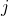which tile all of phase space. That is, every phase point (configuration and set of velocities if you wish) is in some cell but no phase point is in more than one cell. We’ll also assume that the initial and target states, A and B, consist exactly of non-overlapping sets of these phase-space cells. Ifis the probability (fractional occupancy) of cellin the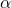= A-to-B steady state, with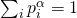and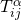is the conditional probability to transition fromtoin “lag” timein thesteady state, then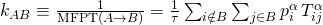This looks messy at first but it’s just a way of counting all the probability flow into state B in the A-to-B steady state, where the flow fromtois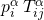in time.

We’ll derive the discrete relation by working backward from the steady state itself. Note that we’re not proving the (continuous) Hill relation itself – that’s already done. We’re assuming the continuous form is true, and we will assume we have complete knowledge of the steady state. You can imagine we have a trillion copies of our system running independently and that together these systems constitute a steady state in which systems reaching B are re-initiated at A (according to our chosen distribution within A).

So we’ve got a lot of information in our hands. We just want to make sure it fits together the right way. And, like I said, I hope you’ll agree all this is obvious … in the end.

Let’s first “generate” the transition probabilitiesfrom the trillion copies of our system. We examine all our systems, and of those that are in cellat a given time, we count the fraction that are found in cellat time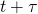. We average this fraction over all the systems and over allto obtain, which therefore depends on the chosen “lag” time.

Critically, this transition probabilitywill depend on the intra-cell distribution. For example, in thesteady state, perhaps more trajectories are closer to one edge of the cell than another.As the simplest possible instance, consider the case of simple diffusion in one dimension, as sketched above. In thesteady-state, the density of trajectories decreases linearly as B is approached. Therefore the probability to transition from any cellis lower to the subsequent cellcloser to B than to the preceding cell! Importantly, this distribution is different from the equilibrium distribution, which would be used in a standard Markov state model, and which is simply constant in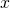.

Moving on. Now that we have the, we must obtain thevalues. We can do this in two ways. Most simply, we can simply calculate the fractional occupancies from our ensemble of systems in thesteady state. Alternatively, we could use the set ofvalues within a linear algebra (matrix) formulation to calculate the, so long as we appropriately set up the source and sink boundary conditions. In any case, we can certainly obtain the steady-state probabilities.

Now let’s recall our actual goal – to calculate the probability flow per second into target state B, which is equivalent to the rate (inverse MFPT) according to the continuous Hill relation. Our reference value, which is correct by definition, is just the fraction of all trajectories which newly arrive to B when we examine the ensemble every.

We want to obtain this same fraction of newly arriving trajectories from the set ofandvalues. Let’s consider just one pair of cellsand, whereis part of B andis not.gives the conditional probability to arrive in B after ainterval, but we’re interested in the actual probability which will arrive. Well, we know thatcontains probabilityat all times in steady state. Thus, the actual probability arriving from cellto cellwill bebecausegives the fraction of the cellprobability which will make the transition.

Now the discrete Hill relation is simply a sum of the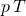terms over all cellswhich transition to cells within B. We don’t have to consider any cells which only lead to indirect transitions to B, perhaps over multipleintervals: after all, everything which makes an indirect transition ultimately makes a direct transition – and we’re including all the direct transitions at every time.

To summarize, the discrete Hill relation is correct whenevervalues are correct, and those in turn will be unbiased when calculated based on the steady-state distribution of trajectories within every cell. That is, the fundamental transition probabilities must be estimated from a specific initial distribution within each cell that corresponds to the steady-state of interest. The equilibrium distribution is wrong in this case, and that shouldn’t be surprising given that we’re calculating a non-equilibrium quantity.

That’s it! I hope in the end all this seems obvious … but hopefully it wasn’t a total waste of your time!!